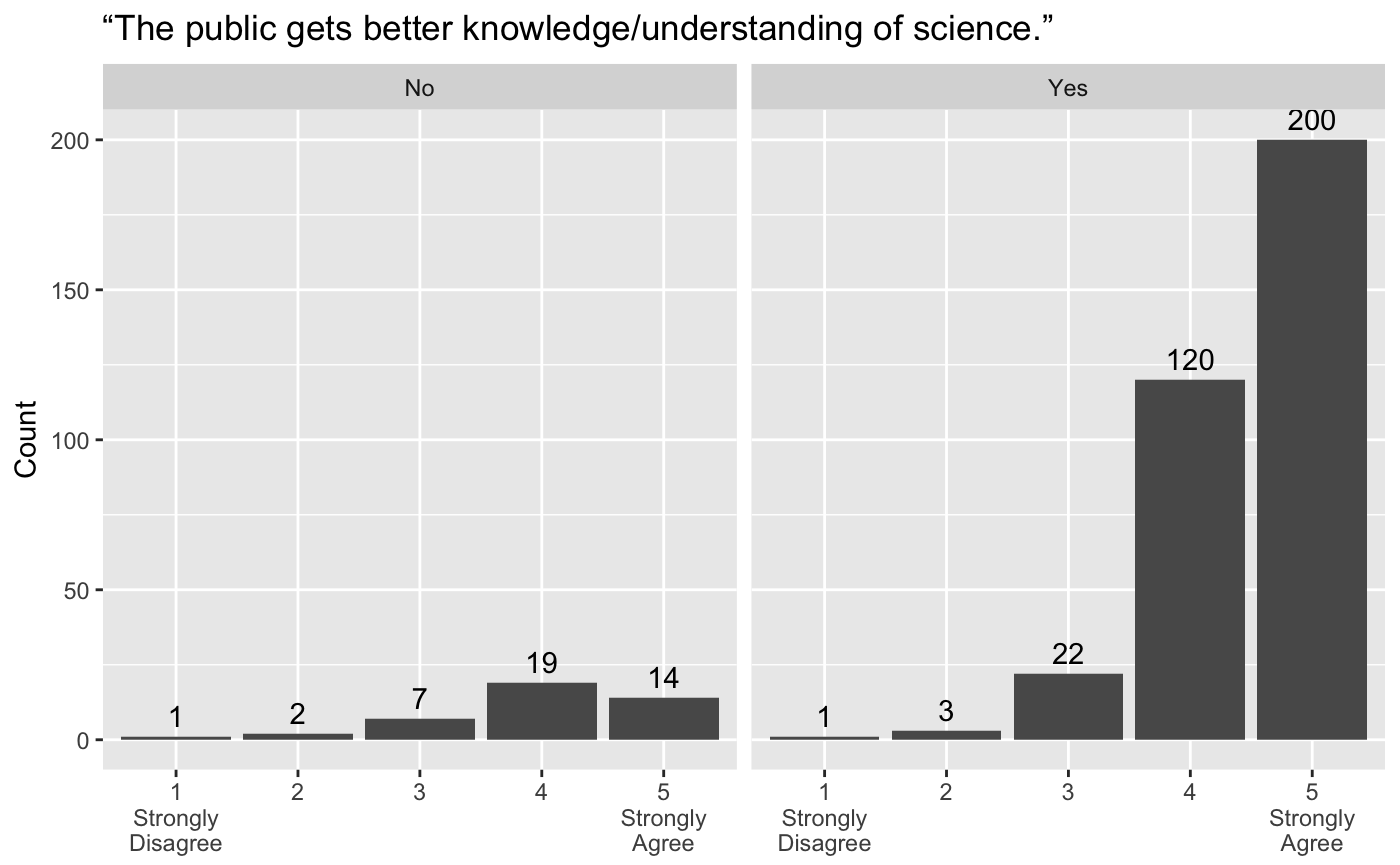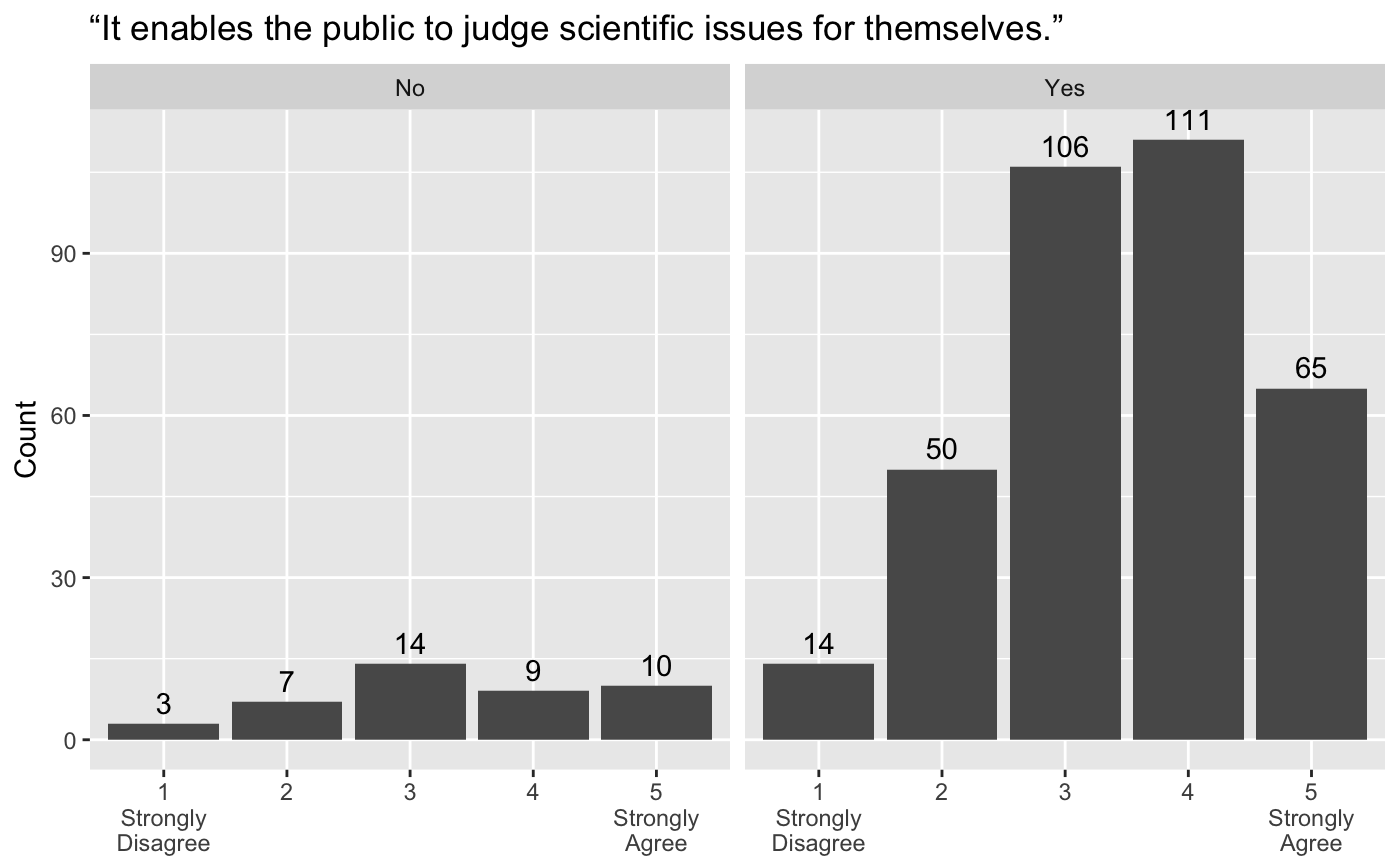## Intro

The survey presented some questions concerning the benefits of doing outreach, both to the researcher themself and to wider society. Here, we take a look at the ratings for these benefits in a bit of detail.

``````# NOTE: This dataset is still private and so this data frame is generated
# from within the storage environment of the file in question.
library(dplyr, warn.conflicts = FALSE)
library(gridExtra, warn.conflicts = FALSE)
library(ggplot2, warn.conflicts = FALSE)
library(magrittr, warn.conflicts = FALSE)
# for long form conversion of data frame
library(reshape2)``````

The responses are split into two categories based on whether the respondents had NOT participated in outreach previously (“No”) or whether they had (“Yes”). Responses with “Not applicable” have been removed from the datasets plotted below.

## Benefits to society

Below, we see responses to questions concerning one of the benefits to society: namely, “The public gets better knowledge/understanding of science.”

``````ggplot(dat, aes(x = factor(better_knowledge_science), y = ..count..)) +
geom_bar(stat = "count") +
geom_text(stat = "count", aes(label = ..count..,
y=..count..),
vjust=-0.5) +
facet_grid(. ~ previous_participation) +
scale_x_discrete(name = NULL,
limits = c(1:5),
drop = FALSE,
labels = c("1" = "1\nStrongly\nDisagree",
"2" = "2", "3" = "3", "4" = "4",
"5" = "5\nStrongly\nAgree")) +
ylab("Count") +
labs(title = "“The public gets better knowledge/understanding of science.”")``````Similarly, we see the responses for the remaining eight questions concerning societal benefits.

``````ggplot(dat, aes(x = factor(judge_scientific_issues), y = ..count..)) +
geom_bar(stat = "count") +
geom_text(stat = "count", aes(label = ..count..,
y=..count..),
vjust=-0.5) +
facet_grid(. ~ previous_participation) +
scale_x_discrete(name = NULL,
limits = c(1:5),
drop = FALSE,
labels = c("1" = "1\nStrongly\nDisagree",
"2" = "2", "3" = "3", "4" = "4",
"5" = "5\nStrongly\nAgree")) +
ylab("Count") +
labs(title = "“It enables the public to judge scientific issues for themselves.”")````````````ggplot(dat, aes(x = factor(lives_informed_decisions), y = ..count..)) +
geom_bar(stat = "count") +
geom_text(stat = "count", aes(label = ..count..,
y=..count..),
vjust=-0.5) +
facet_grid(. ~ previous_participation) +
scale_x_discrete(name = NULL,
limits = c(1:5),
drop = FALSE,
labels = c("1" = "1\nStrongly\nDisagree",
"2" = "2", "3" = "3", "4" = "4",
"5" = "5\nStrongly\nAgree")) +
ylab("Count") +
labs(title = "“It enables the public to make informed decisions about their lives.”")``````